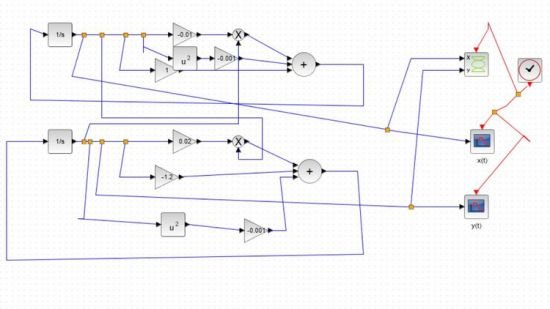﻿ Computer Simulation of Realistic Mathematical Models » GFXhome WS

Information of news
24-06-2021, 16:59

### Computer Simulation of Realistic Mathematical Models

Category: TutorialsMP4 | Video: h264, 1280x720 | Audio: AAC, 44.1 KHz, 2 Ch
Genre: eLearning | Language: English + srt | Duration: 11 lectures (1h 37m) | Size: 986.6 MB

==========

How to model the interaction between prey and predators, as well as the evolution of epidemics
What you'll learn:
How to create and interpret computer simulations of real-life mathematical models using open-source software
How epidemics evolve
How the prey-predator model (or Lotka-Volterra model) explains a variety of real-world phenomena
How the parameters of the model affect results

Requirements
the basics of calculus could be enough, especially what derivatives and functions are.

Description
In this course two mathematical models are analyzed: one is the so-called Lotka-Volterra model, which is also known as prey-predator model, and the other is the model of epidemics. We will analyze and solve these models using free and open-source software called Scilab (quite similar to Matlab). In particular, we will use a tool that is contained in Scilab called Xcos, which will help us construct the mathematical models.

The models presented in the course are very important in applied mathematics because they can explain a variety of phenomena. The Lotka-Volterra model derives its name from the mathematicians who first employed it to explain some real-life phenomena: Lotka used this model to explain the interaction between two molecules, so he was interested in chemical reactions, whereas Volterra was an Italian mathematician who used this model to explain why the number of sharks in the Adriatic Sea had increased substantially during the first world war with respect to the pre-war and the post-war periods.

The discovery about the greater percentage of sharks was made by Volterra's son-in-law, whose name was Umberto D’Ancona. D'Ancona was an Italian biologist who made this observation from the data he collected, and asked Volterra to analyze this problem mathematically, knowing that Volterra was a respected mathematician. Volterra took the challenge and decided to create a mathematical model, which is now known as the Lotka-Volterra model, or prey-predator model. This model focuses on the interaction between two populations: a population of prey versus a population of predators. In this case, the population of predators is represented by sharks, whereas the population of prey is represented by prey-fish.

Volterra understood that the reason why the number of sharks increased dramatically during the first world war was due to the less intense activity of fishing, which had interfered with the interaction between sharks and prey-fish.

We are going to see this more thoroughly in the course; besides, the prey-predator model can be used to explain other interesting phenomena that I will mention at the end of the first part of the course. After that, we are going to study epidemics and we will use the same concepts previously introduced with Scilab.

The mathematics that we need in the course are not difficult; you just need to know what derivatives and functions are, but we are not going into the mathematical details of how to solve a model. In fact, I want to focus more on the practical applications.

Who this course is for
Students who want to understand how to implement mathematical models using Scilab
Students who want to understand how to construct a mathematical model

or
https://rapidgator.net/file/f7b9edec061c58d69f7262f58b723033/Computer_Simulation_of_Realistic_Mathematical_Models.rar.html[/QUOTE]Site BBcode/HTML Code:
Dear visitor, you went to the site as unregistered user.